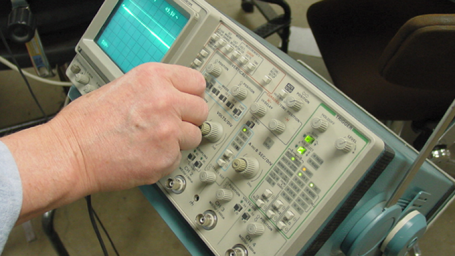Interactive

# Evaluating Series and Parallel DC Circuit Performance

Martech
Updated Jan 21, 2021

After this lesson, you will be able to describe how current, voltage, resistance, and power flow through series and parallel DC circuits. You will also be able to calculate values of current, voltage, resistance, and power flow in DC series and parallel circuits. Finally, you will be able to explain some techniques for evaluating DC circuit performance. • Explain the manner current flows through DC series and parallel circuits • Describe the method of determining voltage in DC series and parallel circuits • Explain calculating resistance in DC series circuits • Describe the calculations of power in DC series and parallel circuits • Explain some techniques for evaluating DC circuit performance

;